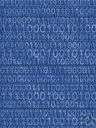# binary number system

Also found in: Thesaurus, Financial, Acronyms, Encyclopedia.

## binary number system

A method of representing numbers that has 2 as its base and uses only the digits 0 and 1. Each successive digit represents a power of 2. For example, 10011 represents (1 × 24) + (0 × 23) + (0 × 22) + (1 × 21) + (1 × 20), or 16 + 0 + 0 + 2 + 1, or 19.
 Noun 1binary number system - a positional system of numeration that uses binary digits and a radix of twopositional notation, positional representation system - a numeration system in which a real number is represented by an ordered set of characters where the value of a character depends on its position